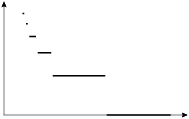arminstraub.com

# Step Functions

## SimplyDraw - Step FunctionsThis example takes advantage of a feature which is not yet found in PyX: a graph style for plotting step functions. Just use it as a replacement for `style.line` when you know that your graph will be a step function.

It would certainly be nice if `style.steps` could handle arbitrary discontinuous graphs, but this has yet to be implemented in a nice manner.

```g = mathxy(height=4,
x=axis.linear(min=0, max=3.3, parter=None),
y=axis.linear(min=0, max=2, parter=None))

g.plot(data.function(lambda e: log(floor(exp(e**-.6))),
min=0.001, max=3),
[style.steps([linewidth.THick, linestyle.solid])])

insert(g)
```